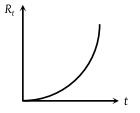NEET Physics Current Electricity Questions Solved

The resistance Rt of a conductor varies with temperature t as shown in the figure. If the variation is represented by ${R}_{t}={R}_{0}\left[1+\alpha t+\beta {t}^{2}\right]$, then(1) α and β are both negative

(2) α and β are both positive

(3) α is positive and β is negative

(4) α is negative and β are positive

Explanation is a part of a Paid Course. To view Explanation Please buy the course.

Difficulty Level: